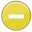#equation 中文解釋 wordnet sense Collocation Usage Collins Definition
Noun
/iˈkwāZHən/,Font size:equations, plural;
1. A statement that the values of two mathematical expressions are equal (indicated by the sign =)

2. The process of equating one thing with another
• - the equation of science with objectivity
3. A situation or problem in which several factors must be taken into account
• - money also came into the equation
4. A symbolic representation of the changes that occur in a chemical reaction, expressed in terms of the formulae of the molecules or other species involved

1. a mathematical statement that two expressions are equal
2. equality: a state of being essentially equal or equivalent; equally balanced; "on a par with the best"
3. the act of regarding as equal
4. An equation is a mathematical statement that asserts the equality of two expressions. Equations consist of the expressions that have to be equal on opposite sides of an equal sign, as in
5. "Equation" is one of the most original and intriguing poems of Dr Tapan Kumar Pradhan, the Indian writer and poet, and it won Kendriya Sahitya Akademi's Indian Literature Golden Jubilee Award for Poetry in 2007. ...
6. This is a list of episodes of the science fiction television series Fringe, which premiered on Fox on September 9, 2008. ...
7. (Equational) Equative is a case with the meaning of comparison, or likening. The equative case has been used in very few languages in history. It was used in the Sumerian language.
8. An assertion that two expressions are equal, expressed by writing the two expressions separated by an equal sign; from which one is to determine a particular quantity; A small correction to observed values to remove the effects of systematic errors in an observation
9. An equations module integrated with Motion. This module enables you to use Motion models in independent simulation programs.
10. An equation is a math sentence that says that 2 things are equal. An equation always has an equal (=) sign. The thing or things that are on the left side of the equal sign are equal to the things on the right side of the equal sign. ...
11. a mathematical sentence containing an equal sign.
12. Within Netica, the probabilistic or deterministic relationship between nodes may be expressed using an equation, as an alternative to CPT or function tables. ...
13. A set of properties, their intended actions and reactions with or without a given goal, and a possible and achievable solution if a goal is a presented.
14. An equation is those points on the highway centerline in which it is necessary to make one station number equal to another station number.
15. A grouping of terms or values that use a sign to show an equal relationship — that two quantities are equal. By using an equation, tough problems can be reduced to easier problems and simpler answers.
16. A mathematical statement in which equal values (or the mathematical statements producing the values) appear to the right and left of an equal sign. ...
17. relationship between the assets, liabilities and equity, where Assets=Liabilities+Owners' Equity.
18. a math statement showing that two things are equal; It is indicated by this sign = . An equation is a statement of equality existing between numbers or combinations of numbers. An algebraic statement connected by an equal sign.
19. Creates a mathematical relation between sketch dimensions, using dimension names as variables, or between feature parameters, such as the depth of an extruded feature or the instance count in a pattern.
20. The act or process of equating or of being equated.
21. A representation of a chemical reaction, usually written as a linear array in which the symbols and quantities of the reactants are separated from those of the products by an equal sign, an arrow, or a set of opposing arrows.^
22. a balanced relationship between numbers and/or symbols. A mathematical sentence.
23. Equations describe the relations between the dependent and independent variables. An equal sign "=" is required in every equation.